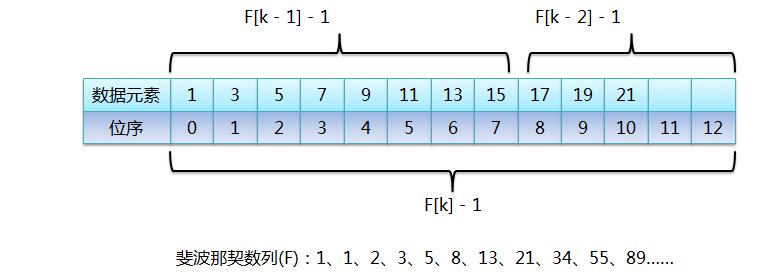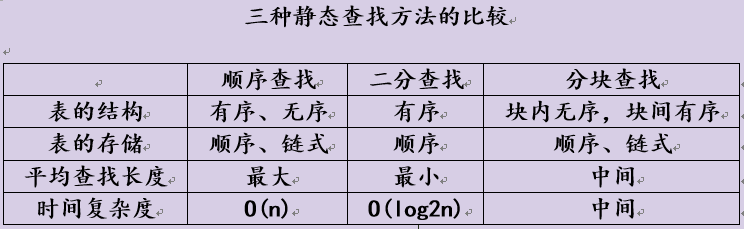# 查找算法总结及其算法实现（Python/Java）算法 同时被 3 个专栏收录20 篇文章 0 订阅# 前言

• 查找算法的定义和思路，动画演示
• 查找算法的代码实现：Python和Java
• 查找算法性能分析：时间空间复杂度分析
• 不同排序算法最佳使用场景

# 预备知识

## 查找算法分类

1）静态查找和动态查找；

2）无序查找和有序查找。

• 无序查找：被查找数列有序无序均可；
• 有序查找：被查找数列必须为有序数列。

## 平均查找长度（Average Search Length，ASL）

Pi：查找表中第i个数据元素的概率。

Ci：找到第i个数据元素时已经比较过的次数。

## 查找性能

• 顺序查找，时间复杂度O(N),
• 分块查找，时间复杂度O(logN+N/m);
• 二分查找，时间复杂度O(logN)
• Fibonacci查找，时间复杂度O(logN)
• 差值查找，时间复杂度O(log(logN))
• 哈希查找，时间复杂度O(1)

# 查找算法

## 1. 顺序查找

（假设每个数据元素的概率相等） ASL = 1/n(1+2+3+…+n) = (n+1)/2 ;

Java实现：

public static int SequenceSearch(int a[], int value, int n) {
for(int i=1;i<n;i++) {
if(a[i]==value) {
return i;
}
}
return -1;
}


### 2.二分查找

Java实现：

// 迭代版
public static int BinarySearch(int a[], int value, int n) {
int low = 0;
int high = n-1;
int mid = 0;
while (low <= high) {
mid = low + (high - low) * 1/2;
if(a[mid] == value) {
return mid;
}
if(a[mid] > value) {
high = mid - 1;
}
else {
low = mid + 1;
}
}
return -1; //return low 应该插入的位置
}
// 递归版
public int binarySearchRecur(int[] nums, int target, int low, int high) {
if (low > high) { return low; } //base case
int mid = low + (high - low) / 2;
if (nums[mid] > target) {
return binarySearchRecur(nums,target,low,mid-1);
} else if (nums[mid] < target) {
return binarySearchRecur(nums,target,mid+1,high);
} else {
return mid;
}
}
//含有重复数字的情况
//主要区别：等于mid也要将high-1；直到low>high结束，返回low（其实是高位，重复数字的第一位）
public static int BinarySearchDuplicate(int a[], int value, int n) {
int low = 0;
int high = n-1;
int mid = 0;
while (low <= high) {
mid = low + (high - low) * 1/2;
if(a[mid] >= value) {
high = mid - 1;
}
else {
low = mid + 1;
}
}
return low;
}
//含有重复数字：递归版
public int firstOccurrenceRecur(int[] nums, int target, int low, int high) {
if (low > high) { return low; }
int mid = low + (high - low) / 2;
if (nums[mid] < target) {
return firstOccurrenceRecur(nums,target,mid + 1,high);
} else {
return firstOccurrenceRecur(nums,target,low,mid-1);
}
}


### 3.插值查找

mid=low+(high-low)*(key-a[low])/(a[high]-a[low])//(1/2)换为(key-a[low])/(a[high]-a[low])


Java实现：

public static int InsertionSearch(int a[], int value, int n) {
int low = 0;
int high = n-1;
int mid = 0;
while (low <= high) {
mid = low + (high-low) * (value-a[low]) / (a[high]-a[low]);
if(a[mid] == value) {
return mid;
}
if(a[mid] > value) {
high = mid - 1;
}
else {
low = mid + 1;
}
}
return -1;
}


### 4. 斐波那契查找

https://blog.csdn.net/zsw12013/article/details/50003505Java：

public final static int MAXSIZE = 20; // fib length
public static int[] fibonacci() {
int[] f = new int[MAXSIZE];
int i = 0;
f = 1;
f = 1;
for (i = 2; i < MAXSIZE; i++) {
f[i] = f[i - 1] + f[i - 2];
}
return f;
}

public static int fibonacciSearch(int[] a, int value, int n) {
int low = 0;
int high = n - 1;
int mid = 0;

// 斐波那契分割数值下标
int k = 0;
// 序列元素个数
int i = 0;
// 获取斐波那契数列
int[] f = fibonacci();
// 获取斐波那契分割数值下标
while (n > f[k] - 1) {
k++;
}

// 创建临时数组
int[] temp = new int[f[k] - 1];
for (int j = 0; j < n;j++) {
temp[j] = a[j];
}

// 序列补充至f[k]个元素
// 补充的元素值为最后一个元素的值
for (i = n; i < f[k] - 1; i++) {
temp[i] = temp[high];
}

//        for (int j : temp) {
//            System.out.print(j + " ");
//        }
//        System.out.println();

while (low <= high) {
// low：起始位置
// 前半部分有f[k-1]个元素，由于下标从0开始
// 则-1 获取 黄金分割位置元素的下标
mid = low + f[k - 1] - 1;

if (temp[mid] > value) {
// 查找前半部分，高位指针移动
high = mid - 1;
// （全部元素） = （前半部分）+（后半部分）
// f[k] - 1 = f[k-1] -1 + f[k-2] -1
// 因为前半部分有f[k-1]个元素，所以 k = k-1
k = k - 1;
} else if (temp[mid] < value) {
// 查找后半部分，高位指针移动
low = mid + 1;
// （全部元素） = （前半部分）+（后半部分）
// f[k] -1 = f[k-1] -1 + f[k-2] - 1
// 因为后半部分有f[k-2]个元素，所以 k = k-2
k = k - 2;
} else {
// 如果为真则找到相应的位置
if (mid <= high) {
return mid;
} else {
// 出现这种情况是查找到补充的元素
// 而补充的元素与high位置的元素一样
return high;
}
}
}
return -1;
}


Python：

MAXSIZE = 20

def fibonacci():  # 1, 1, 2, 3, 5, 8, 13, 21, 34, 55, 89
f =  * MAXSIZE
f = 1
f = 1
for i in range(2, MAXSIZE):
f[i] = f[i-1] + f[i-2]
return f

def fibonacciSearch(array, value):
low, mid, high = 0, 0, len(array)-1
k = 0
f = fibonacci()
while len(array) > f[k]-1:
k += 1
temp = array + [array[-1] * (f[k]-1-len(array))]
while low <= high:
mid = low + f[k-1] - 1
if temp[mid] > value:
high = mid - 1
k = k - 1
elif temp[mid] < value:
low = mid + 1
k = k - 2
else:
if mid <= high: # 如果在high位前，则返回mid位置，否则返回high位置
return mid
else:
return high
return -1

if __name__ == '__main__':
a = [1, 3, 5, 6, 7, 88]
print(fibonacciSearch(a, 2))



### 5.树表查找

#### 5.1 最简单的树表查找算法——二叉树查找算法

1）若任意节点的左子树不空，则左子树上所有结点的值均小于它的根结点的值；

2）若任意节点的右子树不空，则右子树上所有结点的值均大于它的根结点的值；

3）任意节点的左、右子树也分别为二叉查找树。

#### 5.2 平衡查找树之2-3查找树（2-3 Tree）

https://riteme.github.io/blog/2016-3-12/2-3-tree-and-red-black-tree.html

2-3查找树定义：和二叉树不一样，2-3树运行每个节点保存1个或者两个的值。对于普通的2节点(2-node)，他保存1个key和左右两个自己点。对应3节点(3-node)，保存两个Key，2-3查找树的定义如下：

1）要么为空，要么：

2）对于2节点，该节点保存一个key及对应value，以及两个指向左右节点的节点，左节点也是一个2-3节点，所有的值都比key要小，右节点也是一个2-3节点，所有的值比key要大。

3）对于3节点，该节点保存两个key及对应value，以及三个指向左中右的节点。左节点也是一个2-3节点，所有的值均比两个key中的最小的key还要小；中间节点也是一个2-3节点，中间节点的key值在两个跟节点key值之间；右节点也是一个2-3节点，节点的所有key值比两个key中的最大的key还要大。

2-3查找树的性质：

1）如果中序遍历2-3查找树，就可以得到排好序的序列；

2）在一个完全平衡的2-3查找树中，根节点到每一个为空节点的距离都相同。（这也是平衡树中“平衡”一词的概念，根节点到叶节点的最长距离对应于查找算法的最坏情况，而平衡树中根节点到叶节点的距离都一样，最坏情况也具有对数复杂度。）

2-3树的查找效率与树的高度是息息相关的。#### 5.3 平衡查找树之红黑树（Red-Black Tree）

• 红色节点向左倾斜

• 一个节点不可能有两个红色链接

• 整个树完全黑色平衡，即从根节点到所以叶子结点的路径上，黑色链接的个数都相同。• Java中的java.util.TreeMap,java.util.TreeSet；

• C++ STL中的：map,multimap,multiset；

• .NET中的：SortedDictionary,SortedSet 等。

#### 5.4 B树和B+树（B Tree/B+ Tree）

B树可以看作是对2-3查找树的一种扩展，即他允许每个节点有M-1个子节点。

• 根节点至少有两个子节点

• 每个节点有M-1个key，并且以升序排列

• 位于M-1和M key的子节点的值位于M-1 和M key对应的Value之间

• 其它节点至少有M/2个子节点B+树是对B树的一种变形树，它与B树的差异在于：

• 有k个子结点的结点必然有k个关键码；

• 非叶结点仅具有索引作用，跟记录有关的信息均存放在叶结点中。

• 树的所有叶结点构成一个有序链表，可以按照关键码排序的次序遍历全部记录。

B和B+树的区别在于，B+树的非叶子结点只包含导航信息，不包含实际的值，所有的叶子结点和相连的节点使用链表相连，便于区间查找和遍历。• Windows：HPFS文件系统；

• Mac：HFS，HFS+文件系统；

• Linux：ResiserFS，XFS，Ext3FS，JFS文件系统；

• 数据库：ORACLE，MYSQL，SQLSERVER等中。

### 6. 分块查找step1 先选取各块中的最大关键字构成一个索引表；

step2 查找分两个部分：先对索引表进行二分查找或顺序查找，以确定待查记录在哪一块中；然后，在已确定的块中用顺序法进行查找。

## 附录：

Java完整代码，带有测试用例：

public class test {
public static int SequenceSearch(int a[], int value, int n) {
for(int i=1;i<n;i++) {
if(a[i]==value) {
return i;
}
}
return -1;
}

public static int BinarySearch(int a[], int value, int n) {
int low = 0;
int high = n-1;
int mid = 0;
while (low <= high) {
mid = low + (high - low) * 1/2;
if(a[mid] == value) {
return mid;
}
if(a[mid] > value) {
high = mid - 1;
}
else {
low = mid + 1;
}
}
return -1;
}

public static int BinarySearchDuplicate(int a[], int value, int n) {
int low = 0;
int high = n-1;
int mid = 0;
while (low <= high) {
mid = low + (high - low) * 1/2;
if(a[mid] >= value) {
high = mid - 1;
}
else {
low = mid + 1;
}
}
return low;
}

public static int InsertionSearch(int a[], int value, int n) {
int low = 0;
int high = n-1;
int mid = 0;
while (low <= high) {
mid = low + (high-low) * (value-a[low]) / (a[high]-a[low]);
if(a[mid] == value) {
return mid;
}
if(a[mid] > value) {
high = mid - 1;
}
else {
low = mid + 1;
}
}
return -1;
}

public final static int MAXSIZE = 20; // fib length
public static int[] fibonacci() {
int[] f = new int[MAXSIZE];
int i = 0;
f = 1;
f = 1;
for (i = 2; i < MAXSIZE; i++) {
f[i] = f[i - 1] + f[i - 2];
}
return f;
}

public static int fibonacciSearch(int[] a, int value, int n) {
int low = 0;
int high = n - 1;
int mid = 0;

// 斐波那契分割数值下标
int k = 0;
// 序列元素个数
int i = 0;
// 获取斐波那契数列
int[] f = fibonacci();
// 获取斐波那契分割数值下标
while (n > f[k] - 1) {
k++;
}

// 创建临时数组
int[] temp = new int[f[k] - 1];
for (int j = 0; j < n;j++) {
temp[j] = a[j];
}

// 序列补充至f[k]个元素
// 补充的元素值为最后一个元素的值
for (i = n; i < f[k] - 1; i++) {
temp[i] = temp[high];
}

//        for (int j : temp) {
//            System.out.print(j + " ");
//        }
//        System.out.println();

while (low <= high) {
// low：起始位置
// 前半部分有f[k-1]个元素，由于下标从0开始
// 则-1 获取 黄金分割位置元素的下标
mid = low + f[k - 1] - 1;

if (temp[mid] > value) {
// 查找前半部分，高位指针移动
high = mid - 1;
// （全部元素） = （前半部分）+（后半部分）
// f[k] - 1 = f[k-1] -1 + f[k-2] -1
// 因为前半部分有f[k-1]个元素，所以 k = k-1
k = k - 1;
} else if (temp[mid] < value) {
// 查找后半部分，高位指针移动
low = mid + 1;
// （全部元素） = （前半部分）+（后半部分）
// f[k] -1 = f[k-1] -1 + f[k-2] - 1
// 因为后半部分有f[k-2]个元素，所以 k = k-2
k = k - 2;
} else {
// 如果为真则找到相应的位置
if (mid <= high) {
return mid;
} else {
// 出现这种情况是查找到补充的元素
// 而补充的元素与high位置的元素一样
return high;
}
}
}
return -1;
}

public static void main(String[] args) {
int[] a = {1,5,2,7,3,9};
int[] b = {1,3,5,6,7,88};
int[] c = {1,3,5,7,7,88};//带重复元素
int value = 5;
int n1 = a.length;
int n2 = b.length;
int n3 = c.length;
//    	int result = SequenceSearch(a, value, n1);
//    	int result = BinarySearch(b, value, n2);
//    	int result = BinarySearchDuplicate(c, value, n3);
//    	int result = InsertionSearch(b, value, n2);
int result = fibonacciSearch(b, value, n2);
System.out.println(result);
}
}


# 主要参考网页

http://www.cnblogs.com/maybe2030/p/4715035.html#_label6

# 关注我

Github：https://github.com/qqxx6661

### 原创博客主要内容

• 笔试面试复习知识点手册
• Leetcode算法题解析（前150题）
• 剑指offer算法题解析
• Python爬虫相关技术分析和实战
• 后台开发相关技术分析和实战

1. Csdn

http://blog.csdn.net/qqxx6661

• Leetcode题解（Java/Python）
• Python爬虫实战
• Java程序员知识点复习手册

2. 知乎

https://www.zhihu.com/people/yang-zhen-dong-1/

• Java程序员面试复习手册
• LeetCode算法题详解与代码实现
• 后台开发实战

3. 掘金

https://juejin.im/user/5b48015ce51d45191462ba55

4. 简书

https://www.jianshu.com/u/b5f225ca2376

### 个人公众号：Rude3Knife06-08832512-19160
09-18386
08-06175
08-092343
10-13719
07-23105
03-01647
02-04181
05-173883
12-241029
11-215446
02-022754
07-09543
08-01166
04-037234
10-214583
08-26419
11-04290
©️2021 CSDN 皮肤主题: Age of Ai 设计师:meimeiellie点击重新获取扫码支付1.余额是钱包充值的虚拟货币，按照1:1的比例进行支付金额的抵扣。
2.余额无法直接购买下载，可以购买VIP、C币套餐、付费专栏及课程。余额充值